GRE Subject Test: Chemistry : Atomic Radius

Example Questions

← Previous 1

Example Question #1 : Atomic Radius

Which of the following atoms has the largest atomic radius?

S

P

Se

As

As

Explanation:

Most people clearly understand that atomic radius will increase as you go down the periodic table. However, going from left to right will actually decrease atomic radius. The reason is that the increased positive charge in the nucleus from the added protons will pull the electrons closer, decreasing the radius. Of the given options, arsenic and selenium are in the lowest row, but arsenic is more to the left. As a result, it has the largest atomic radius.

Example Question #11 : Periodic Trends

Which of the following alkali metals has the greatest atomic radius?

Lithium

Rubidium

Hydrogen

Sodium

Rubidium

Explanation:

The trend for atomic radius is to increase going from top to bottom, as additional valence shells are added to the atom. Out of the answer choices, rubidium has the highest energy valence shell.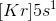With a single electron in the fifth energy level, krypton will have the highest number of energy levels of the group I elements listed.

When moving across a period, atomic radius will decrease as the number of protons increases. These protons increase the attraction between the high-energy electrons and the nucleus, effectively "shrinking" the electron cloud.

Example Question #1 : Atomic Radius

Which of the following has the largest atomic radius?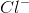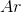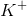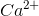Explanation:

Atomic radius increases with increasing effective nuclear charge (Z). Elements toward the right and toward the top of the periodic table have the highest Z values. Protons and electrons are added in pairs as we traverse the periodic table from left to right. A attractive force is established between the positively-charged nucleus and the negatively-charged electron cloud, which increases as the number of particles grows.

When electrons are added or taken away without the same happening to a proton, an imbalance of charge accumulates. When more electrons are present than normal, the electron cloud sags farther away from the nucleus. When fewer electrons are present than normal, the electron cloud is drawn in more tightly toward the nucleus. Atoms with extra electrons (a negative charge) will have larger nuclei than their neutral counterparts. A chloride ion will thus has a larger atomic radius than argon, a potassium ion, or a calcium ion.

Example Question #1 : Atomic Radius

Which of the following elements has the greatest atomic radius?

B

N

F

C

O

B

Explanation:

Atomic radius can be determined using the periodic trends. Atomic radius increases to the left of a period and down a group of the periodic table. Electronegativity, in contrast, increases to the right of a period and up a group of the periodic table. Relating the two, we can see that the greater the atomic radius, the weaker its electronegativity because the electrons are farther away from the nucleus and are unable to feel the attractive force of the protons in the nucleus.

Example Question #1 : Atomic Radius

Which of the given atoms has the smallest atomic radius?

As

N

P

Bi

N

Explanation:

Nitrogen, phosphorous, antimony, and bismuth are all in the same group (column) of the periodic table.

The atomic radius increases from the top of a group to the bottom, due to increased principle shell number (n). As one travels down a group, another s shell is added, meaning that electrons are added in another orbit farther from the nucleus. This serves to increase the atomic radius of the atom.

Example Question #1 : Atomic Radius

Which of the given atoms has the largest atomic radius?

B

Ne

O

Li

Li

Explanation:

Lithium, boron, oxygen, and neon are all in the same row (period) of the periodic table.

The atomic radius decreases from left to right along a period due to increased effective nuclear force. From left to right the atomic number increases, indicating that more protons are added. The addition of protons increases the positive charge in the nucleus, pulling in the outer electrons by increasing the effective nuclear force, decreasing the radius.

In math terms, we can equate effective nuclear force using the force equation between two charged particles.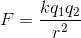We can see that the farther apart the electrons and protons are, the less the force is between them.

Example Question #1 : Atomic Radius

Which of the following would have the greatest atomic radius?

Chlorine

Iodine

Bromine

Flourine

Iodine

Explanation:

Atomic radius increases down each group of the periodic table and toward the left of each period. Since the elements listed are all in the same group, iodine would have the greatest atomic radius because it farther down the period compared to the others.

Example Question #31 : Atoms, Elements, And The Periodic Table

Which of the following correctly describes the trend for atomic radius in the periodic table of elements?

Atomic radius increases with increasing energy level. Atomic radius decreases as new electrons are added within the same orbital.

Atomic radius decreases with increasing energy level. Atomic radius remains constant as new electrons are added within the same orbital.

Atomic radius decreases with increasing energy level. Atomic radius increases as new electrons are added within the same orbital.

Atomic radius increases with increasing energy level. Atomic radius increases as new electrons are added within the same orbital.

Atomic radius increases with increasing energy level. Atomic radius remains constant as new electrons are added within the same orbital.

Atomic radius increases with increasing energy level. Atomic radius decreases as new electrons are added within the same orbital.

Explanation:

Energy level increases moving down a group of the periodic table. As energy level increases, the outer valence shell becomes more distant from the nucleus, causing atomic radius to increase.

Energy level remains constant across a period, but electrons are added within the same orbitals. When new electrons are added within the same orbital, additional protons are also added to the nucleus. This increases the effective nuclear charge, pulling the electrons closer to the nucleus. The trend for atomic radius is to decrease as we move right along a row.

This means that the general trend for atomic radius is to increase as one moves to the left and downward on the periodic table.

Example Question #1 : Atomic Radius

Which atom would be expected to have the largest atomic radius?

Calcium

Nitrogen

Aluminum

Oxygen

Magensium

Calcium

Explanation:

The radius of an atom is determined by the sizes of the orbitals on its outermost shell. Below are the atomic radius trends:

1. Atomic radius increases from top to bottom within each column.

2. Atomic radius decreases from left to right within each period.

Because calcium is located closest to the bottom right on the periodic table, it has the highest atomic radius. Namely, this is due to the fact that calcium's highest energy electron is in the fourth energy shell.

Example Question #1 : Atomic Radius

Which atom would be expected to have the smallest atomic radius?

Nitrogen

Potassium

Phosphorus

Magnesium

Sodium

Nitrogen

Explanation:

The radius of an atom is determined by the sizes of the orbitals on its outermost shell. Below are the atomic radius trends:

1. Atomic radius increases from top to bottom within each column.

2. Atomic radius decreases from left to right within a period.

Because nitrogen is furthest to the the top right on the periodic table, it has the smallest atomic radius.

← Previous 1

All GRE Subject Test: Chemistry Resources